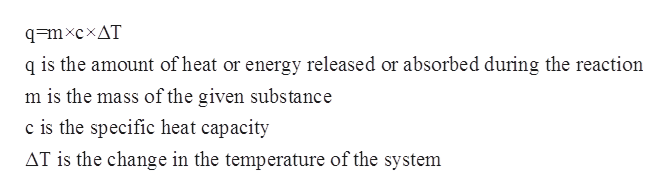# Calculate the energy required to heat 541.0mg of mercury from 11.4°C to 16.6°C. Assume the specific heat capacity of mercury under these conditions is 0.139 J·g−1K−1. Be sure your answer has the correct number of significant digits.

Question
25 views

Calculate the energy required to heat 541.0mg of mercury from 11.4°C to 16.6°C. Assume the specific heat capacity of mercury under these conditions is 0.139 J·g−1K−1. Be sure your answer has the correct number of significant digits.

check_circle

Step 1

The specific heat capacity for a given substance refers to the amount of heat which is needed to raise the temperature of a given substance per unit of its mass. The mathematical relationship between specific heat capacity, mass, change in temperature and the heat of the solution is given by the following equation:help_outlineImage Transcriptioncloseq=mxcxAT q is the amount of heat or energy released or absorbed during the reaction m is the mass of the given substance c is the specific heat capacity AT is the change in the temperature of the system fullscreen
Step 2

The initial temperature is given to be 11.4oC =284.55 K.

The final temperature after is given to be 16.6oC = 289.75 K.

The specific heat capacity of mercury is given to be 0.139 J g-1K-1.

The mass of mercury is given to be 541.0 mg=0.541 g.

The change in the temperature of the system can be calculated as follows:

Step 3

Substituting all the values in the...

### Want to see the full answer?

See Solution

#### Want to see this answer and more?

Solutions are written by subject experts who are available 24/7. Questions are typically answered within 1 hour.*

See Solution
*Response times may vary by subject and question.
Tagged in

### Physical Chemistry